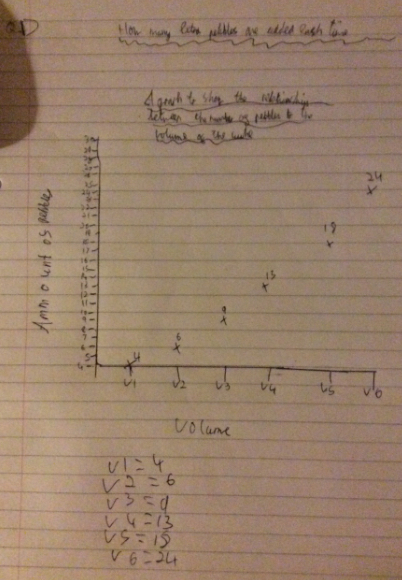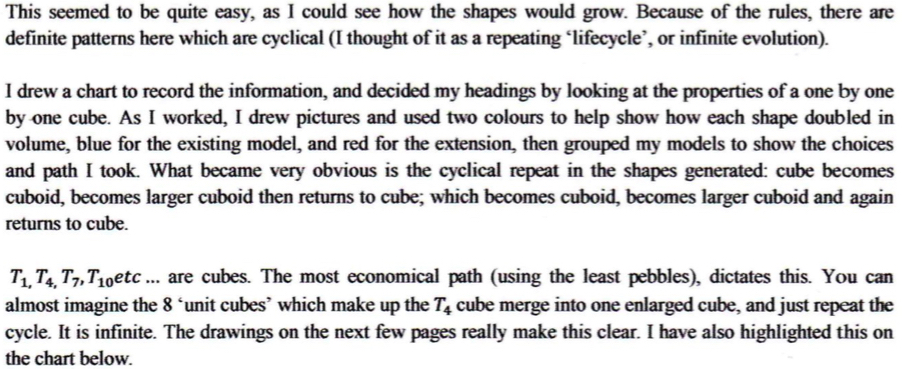#### You may also like### Consecutive Numbers

An investigation involving adding and subtracting sets of consecutive numbers. Lots to find out, lots to explore.### Tea Cups

Place the 16 different combinations of cup/saucer in this 4 by 4 arrangement so that no row or column contains more than one cup or saucer of the same colour.### Exploring Wild & Wonderful Number Patterns

EWWNP means Exploring Wild and Wonderful Number Patterns Created by Yourself! Investigate what happens if we create number patterns using some simple rules.

# More Pebbles

##### Age 7 to 11Challenge Level

Peter from Durham Johnston School wrote:

1) The rule is the difference between the last two numbers in the sequence +1 added onto the previous number = the next number in the sequence.

X = the number you are trying to find, Y and Z = the past two numbers.
Z is the previous number that is before the number you are trying to find.
A = The difference between Z and Y
The difference between Z and Y + 1 = A .
A + 1 + Z = X
To put this into a real situation:
Sequence: 4, 6 , 9 , X
9 - 6 = 3
9 + 3 + 1 = X = 13

This then continues using the same rule. Below is a graph to show the relationship between the volume and amount of pebbles.

2)   The rule is the difference between the previous two numbers in the sequence + 2 + the previous number = the next number in the sequence.
X = The number you are trying to find.
Y and Z = the previous two numbers in the sequence.
The difference between Z and Y = A
A + 2 + Z = X

3) It is always going to be 0.

4) The rule is the same as Q 2.Zach sent in a very thorough explanation.  You can view it in full here: More Pebbles - Zach T.pdf and it starts off like this:Thank you for these solutions to what was really a tough nut to crack. Well done!